• Slides: 10Radial acceleration �Newtons 1 st Law states: �“ An object will remain at rest or continue at a steady speed in a straight line unless acted upon by an unbalanced force. ” �So if an object is moving in a circle, there must be an unbalanced force acting on it, keeping it moving in a circle. therefore it must be accelerating! �Newtons 2 nd Law states: An unbalanced force causes a mass to have a change in its motion. (It will accelerate or change direction) �Remember velocity is a vector. Motion in a circle means its direction is continuously changing.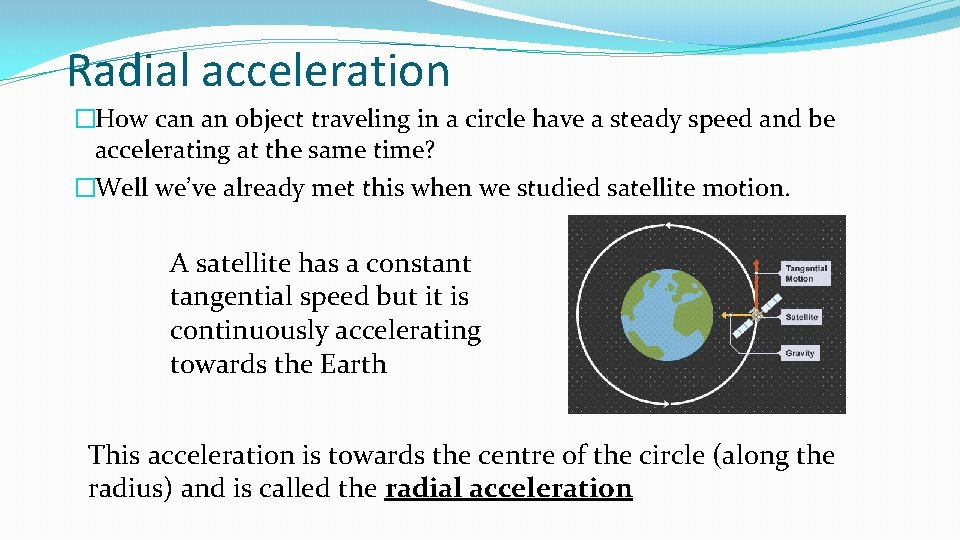Radial acceleration �How can an object traveling in a circle have a steady speed and be accelerating at the same time? �Well we’ve already met this when we studied satellite motion. A satellite has a constant tangential speed but it is continuously accelerating towards the Earth This acceleration is towards the centre of the circle (along the radius) and is called the radial acceleration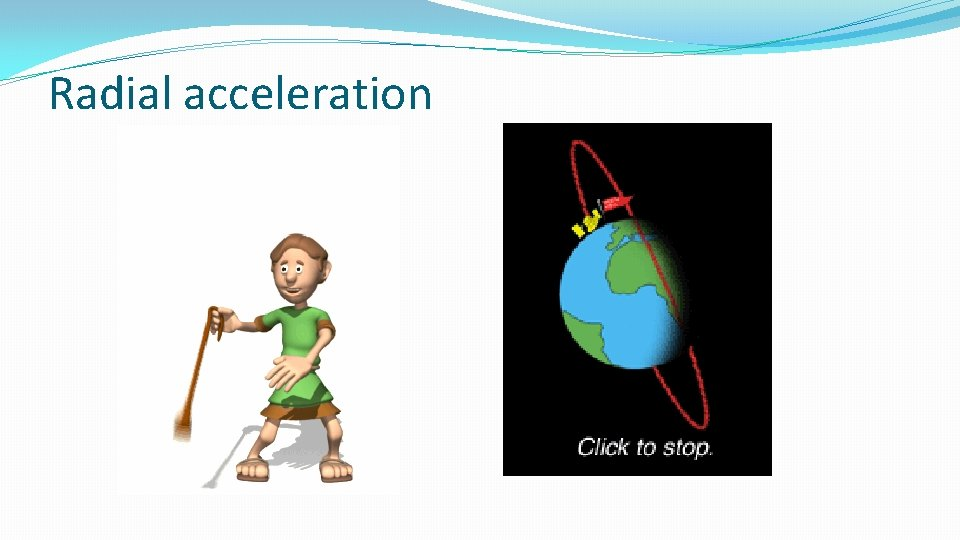Radial acceleration equation �What is the relationship between tangential speed (or angular velocity ) and radial acceleration ? Unfortunately you need to be able to derive this relationship…. so here we go. (but there is very little chance you will be asked this in an exam……)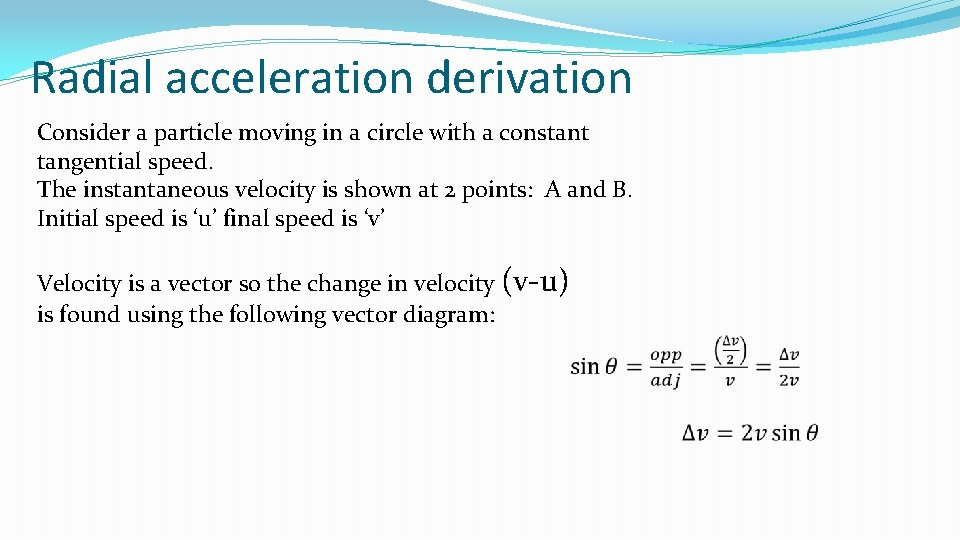Radial acceleration derivation Consider a particle moving in a circle with a constant tangential speed. The instantaneous velocity is shown at 2 points: A and B. Initial speed is ‘u’ final speed is ‘v’ Velocity is a vector so the change in velocity (v-u) is found using the following vector diagram:Radial acceleration derivation (continued) Now we need the Time to go for A B: Now putting both these together…………. For the instantaneous radial acceleration: θ tends to zero, as t tends to zero, therefore; sin θ = θ That’s it……….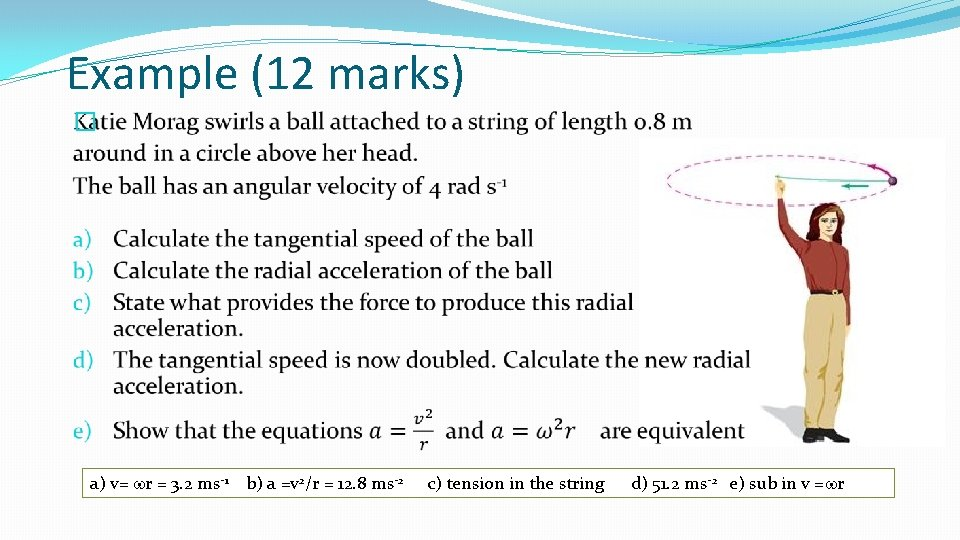Example (12 marks) � a) v= wr = 3. 2 ms-1 b) a =v 2/r = 12. 8 ms-2 c) tension in the string d) 51. 2 ms-2 e) sub in v =wr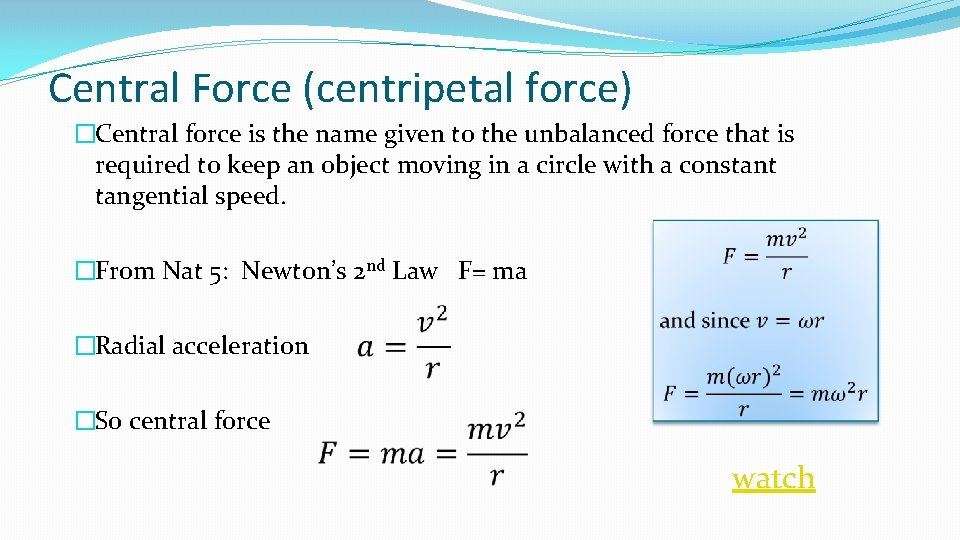Central Force (centripetal force) �Central force is the name given to the unbalanced force that is required to keep an object moving in a circle with a constant tangential speed. �From Nat 5: Newton’s 2 nd Law F= ma �Radial acceleration �So central force watchCentripetal v Centrifugal forces. �Centripetal force is the force required to keep an object moving in a circle. �Centrifugal force is a fictional force. It is not real. It is “what you feel” if you are moving in a circle. You feel as if you are being thrown out of the circle when in fact all your body is doing is trying to continue at a steady speed in a straight line (Newton’s 1 st Law) �What you feel is your reaction to the centripetal force keeping you moving in a circle. �You being “thrown out” of a circular path is only you obeying Newton’s 1 st Law!